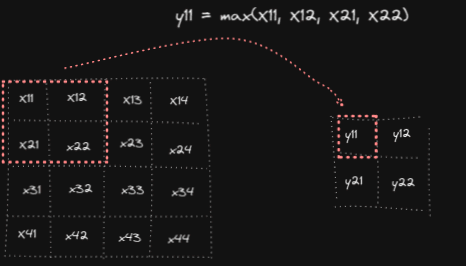# Making Backpropagation, Autograd, MNIST Classifier from scratch in Python

Backpropagation (backward propagation of errors) — is a widely used algorithm in training feedforward networks. It computes the gradient of the loss function with respect to the weights of the network. The main idea of it is to break big functions in small parts and use partial derivatives to get function derivative with using the Chain Rule.

So training the model is basically solving this equation:

And because solving this can be a very hard task, here comes backpropagation and gradient descent(updating weights by a small amount based on the gradient to move in the way of loss minimization).

All this is based on a simple differentiation chain rule:

For example, we have a simple function with 2 nodes(operation):

Let’s say we have 3 variables x=-2, y=5, z=-4, the result will be f=-12, and our target for training is -13. Simple loss=f-target=1.

Now we need to propagate our error(1) back to the x,y,z. To do this we will need to calculate partial derivatives for every function(operation):

And our chain is:

# Let’s build a simple Autograd example

Here we will build a simple automatic differentiation example from the above formulas.

Now let’s see what will happens

As you can see we got the same gradients as in our simple Autograd solution

# Let’s build Neural Networks and solve 2 simple tasks

For simplicity, we will not use Autograd here and will solve a derivative for each layer.

## Classifying MNIST with our custom network

Now let’s solve some classification task on MNIST dataset. We will use some PyTorch utils to make it simpler

And that’s all. Don’t forget that you can run this code in Google Colab

# Support

Become a Patron and support our community to make more interesting articles & tutorials

# Subscribe on New Practical Course

--

--

--

## More from Andrey Nikishaev

Machine Learning and Computer Vision Researcher. Founder LearnML.Today

Love podcasts or audiobooks? Learn on the go with our new app.## Andrey Nikishaev

Machine Learning and Computer Vision Researcher. Founder LearnML.Today

## Multi-Class Image Classification with TensorFlow## Forward and Backward propagation of Max Pooling Layer in Convolutional Neural Networks